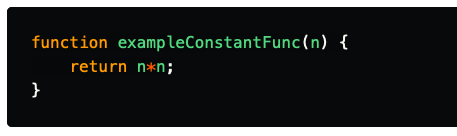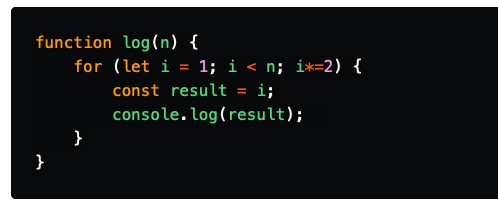‌ ‌ ‌ ‌ ‌ ‌ ‌ ‌ ‌ ‌ ‌ ‌ ‌ ‌ ‌ ‌ ‌ ‌

## Constant time (O(1))

### O(1) does not change with respect to input space. Hence, O(1) is referred to as being constant time. An exmple of an of an O(1):## Linear time (O(n))

### O(n) is linear time and applies to algorithms that must do n operations in the worst-case scenario. most its just A simple basic loop that within it we perform constant time operations. An exmple of an of an O(n):## Logarithmic time O(log(n))

### A Logarithmic time function is one in which the time of execution is proportional to the logarithm of the input size. Consider the following example:## Coefficient Rule: “Get Rid of Constants”

### Every UI developer inevitably runs into situations where they need to make visual### Jama Hassan

Software engineer /Focused Modern UI Technologies